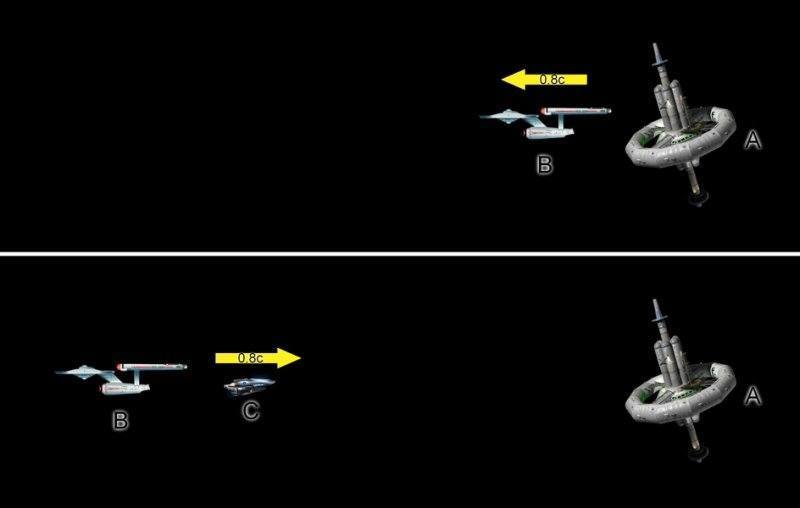I made this pic to illustrate my problem.A space ship (labeled B) departs from a space station (labeled A) at 80% the speed of light (0.8c). A applies the time dilation principle and calculates that B's clocks are running slower than his.

Some time later a smaller craft (labeled C) detaches from B and travels at 80% the speed of light in the opposite direction. B applies the time dilation principle and calculates that C's clocks are running slower than his.

But this results in an illogical situation. B's clocks are slower than A's and C's clocks are slower than B's but C is stationary in A's reference frame so C's clocks are running at the same rate as A's.

What am i doing wrong?